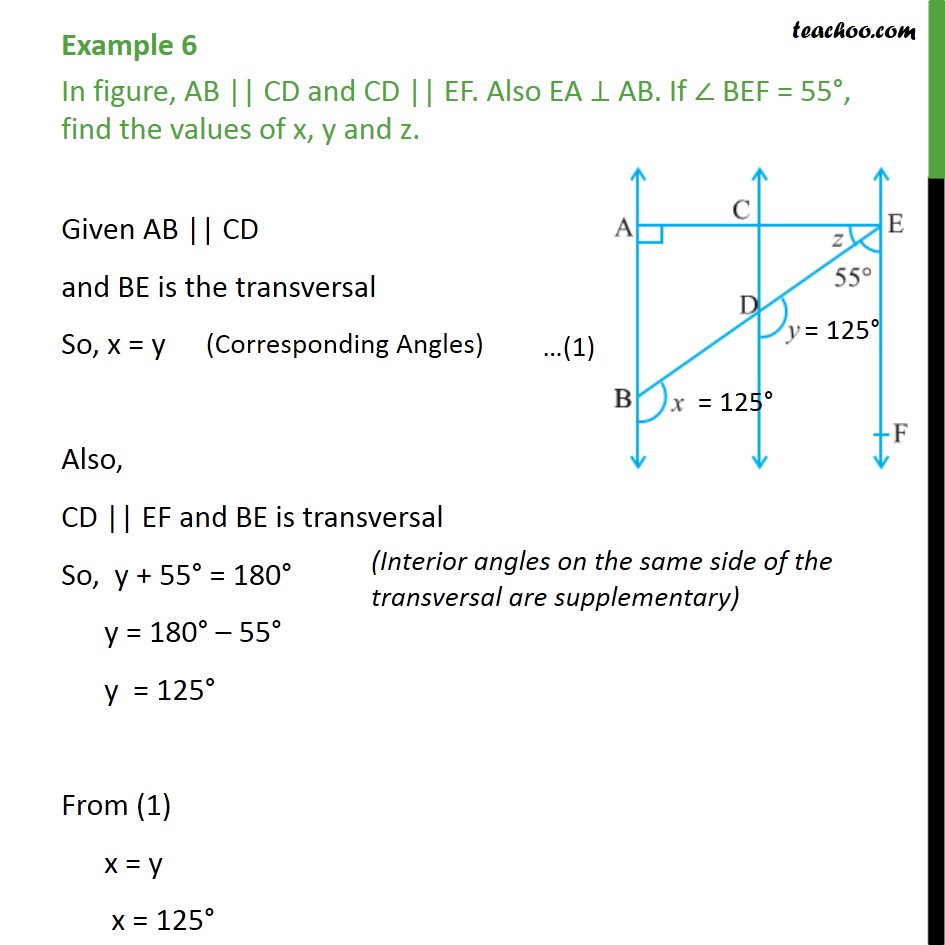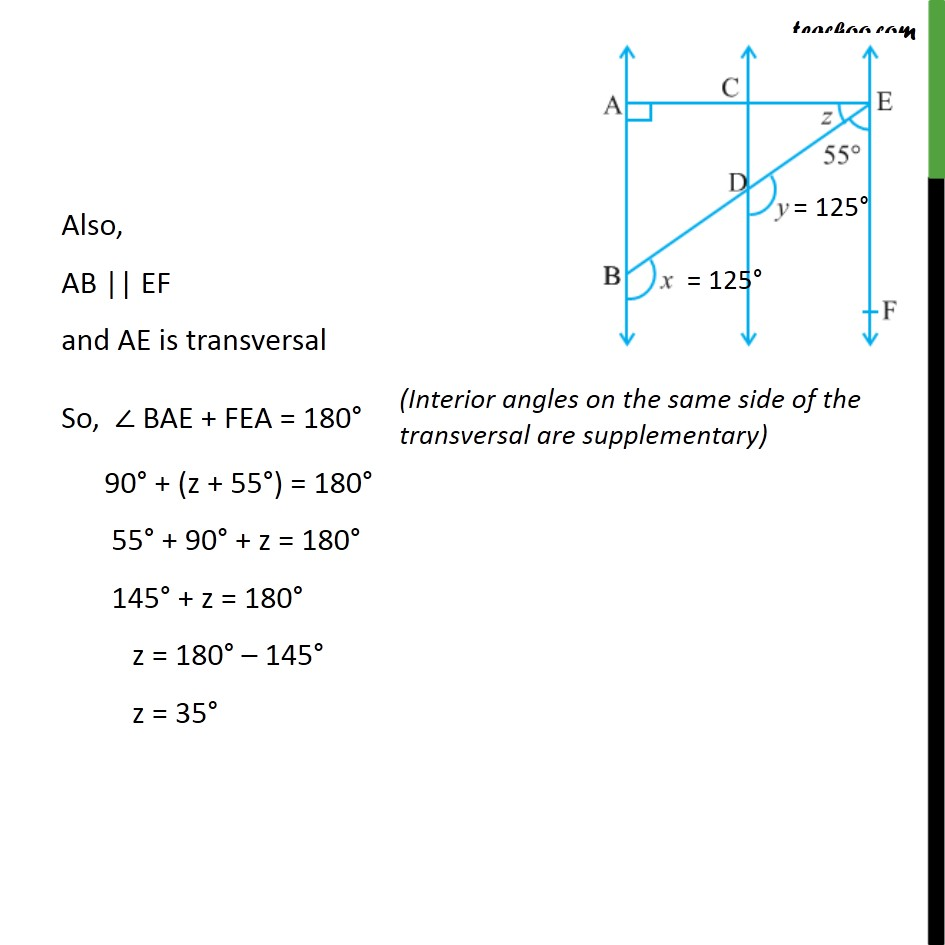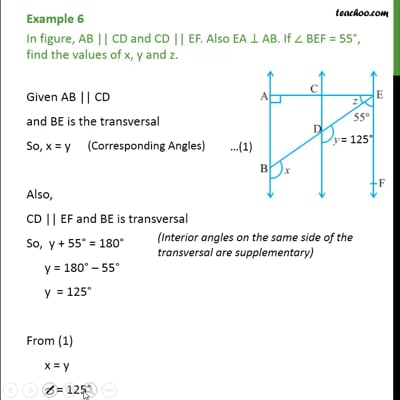Examples

Chapter 6 Class 9 Lines and Angles (Term 1)
Serial order wiseThis video is only available for Teachoo black users

### Transcript

Example 6 In figure, AB || CD and CD || EF. Also EA ⊥ AB. If ∠ BEF = 55°, find the values of x, y and z. Given AB || CD and BE is the transversal So, x = y Also, CD || EF and BE is transversal So, y + 55° = 180° y = 180° – 55° y = 125° From (1) x = y x = 125° Also, AB || EF and AE is transversal So, ∠ BAE + FEA = 180° 90° + (z + 55°) = 180° 55° + 90° + z = 180° 145° + z = 180° z = 180° – 145° z = 35°